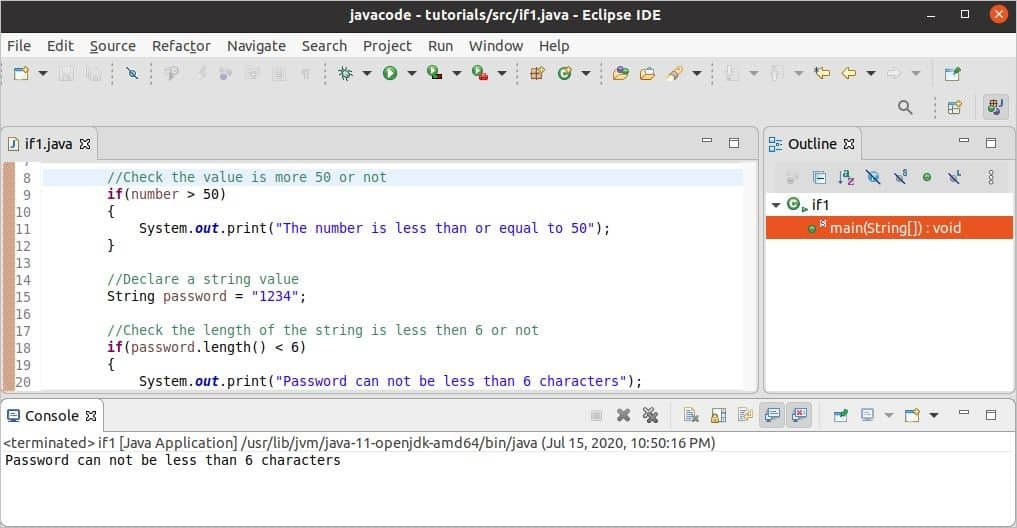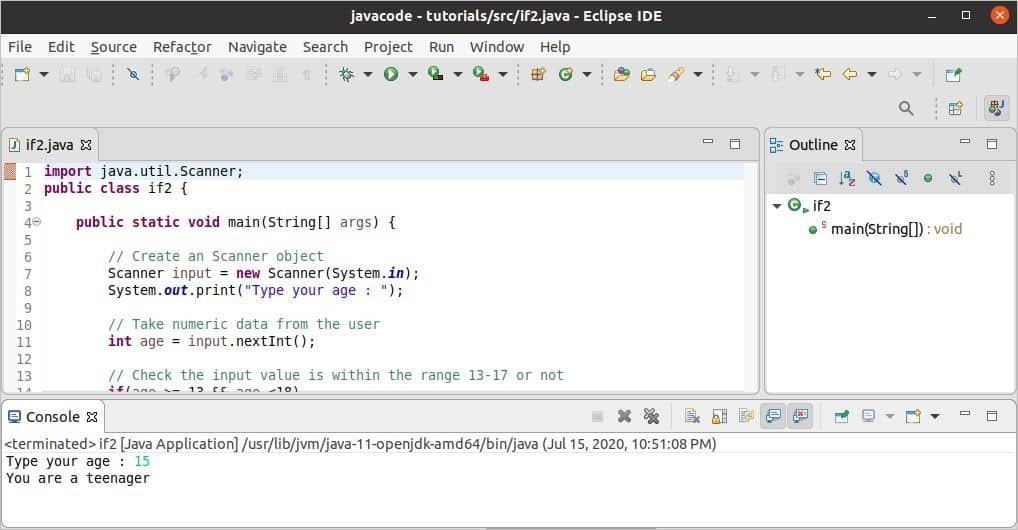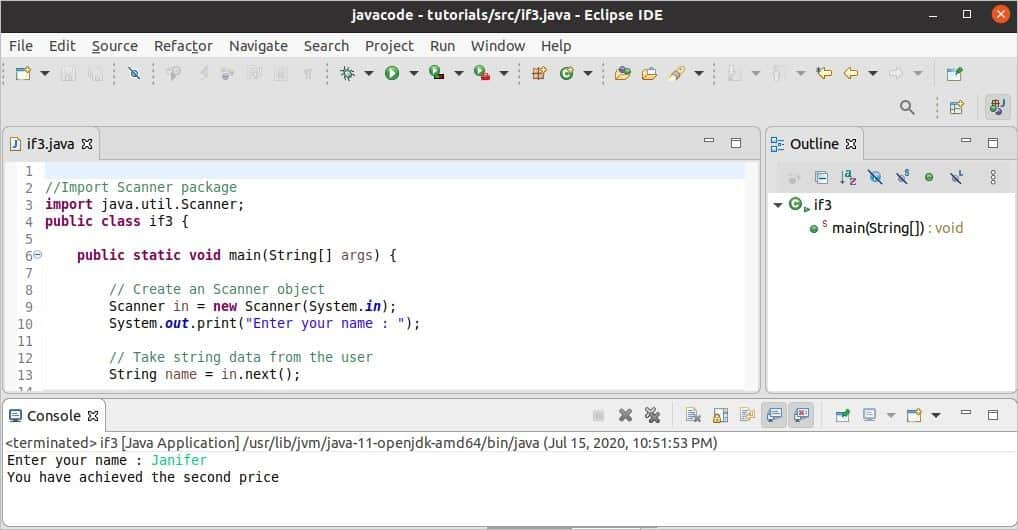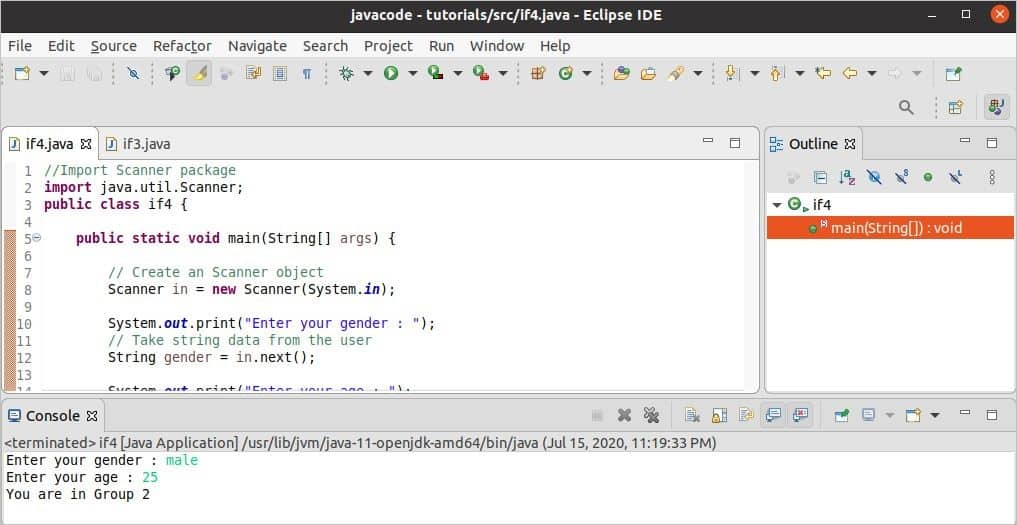Java

# Java if, if-else, if-else-if

The use of a control flow statement is a very common requirement for solving any programming problem. It is mainly used to generate a particular output based on the particular condition. This statement makes the decision based on the Boolean value return by the statement. The declaration of the if-else-if statement is quite similar to other programming languages like C, C++, etc. The uses of different ‘if’ statements in Java are explained in this tutorial.

### Simple ‘if’ statement:

Syntax:

if (conditional expression) {
statement 1…n
}

It checks the conditional expression, and if the expression returns true, then a particular statement(s) will execute otherwise, nothing will execute.

### ‘if-else’ statement:

Syntax:

if (conditional expression) {
statement 1...n
}
else{
statement 1...n
}

If the conditional expression returns true, then a particular statement(s) will execute otherwise other statement(s) will execute.

### ‘if-else-if’ statement:

Syntax:

if (conditional expression 1) {
statement 1...n
}
else if(conditional expression 2) {
statement 1...n
}
.
.
else if(conditional expression n) {
statement 1...n
}
else
statement 1...n

The above ‘if’ statement is also called the ‘if-else-if’ ladder. It checks the first conditional expression, and if it returns false, then it will check the second conditional expression and so on. If all conditional expressions return false, it executes the statement(s) of else part.

### nested ‘if’ statement:

Syntax:

if (conditional expression 1) {
statement 1...n
if (conditional expression 1) {
statement 1...n
}
else
statement 1...n
}

When any ‘if’ statement is declared inside another if statement, then it is called nested ‘if’. If the outer ‘if’ condition returns true, then it will check the inner ‘if’ condition and make decisions based on the return value.

### Example-1: Use of simple ‘if’ statement

The following code shows the simple use of the ‘if’ statement. The first ‘if’ condition checks the value of the number is more than 50 or not. The second ‘if’ condition checks the length of a string is less than 6 or not.

public class if1 {

public static void main(String[] args) {
//Declare a numeric value
int number = 50;

//Check the value is more 50 or not
if(number > 50)
{
System.out.print("The number is less than or equal to 50");
}

//Declare a string value

//Check the length of the string is less then 6 or not
{
System.out.print("Password can not be less than 6 characters");
}
}

}

Output:

The following output will appear after executing the code. Here, the first ‘if’ condition returned false, and no message is printed. The second ‘if’ condition returned true, and a message is printed.### Example-2: Use of ‘if-else’ statement

The following code shows the use of the ‘if-else’ statement. In this code, an integer value is taken from the user. If the input value is between 13 to 17, then ‘if’ condition will return true, a particular message will print otherwise another message will print.

//Import Scanner package
import java.util.Scanner;
public class if2 {

public static void main(String[] args) {

// Create a Scanner object
Scanner input = new Scanner(System.in);

// Take numeric data from the user
int age = input.nextInt();

// Check the input value is within the range 13-17 or not
if(age >= 13 && age <18)
{
System.out.print("You are a teenager");
}
else
{
System.out.print("You are not a teenager");
}

//Close the scanner object
input.close()

}
}

Output:

The following output will appear after executing the code. Here, 15 is taken as input, and the following output is printed because if condition returned true.### Example-3: Use of ‘if-else-if’ statement

The use of the ‘if-else-if’ statement is shown in the following example. Here, a string value will be taken as input from the user. The first ‘if’ condition will check the input value, and if it returns false, then the value will check by the next ‘if’ condition and so on. The message of the else part will print if all ‘if’ conditions return false.

//Import Scanner package
import java.util.Scanner;
public class if3 {

public static void main(String[] args) {

// Create a Scanner object
Scanner in = new Scanner(System.in);

// Take string data from the user
String name = in.next();

// Check the input value equal to 'Jolly' or not
if(name.equals("Jolly"))
{
System.out.print("You have achieved the first price");
}
// Check the input value equal to 'Janifer' or not
else if(name.equals("Janifer"))
{
System.out.print("You have achieved the second price");
}
// Check the input value equal to 'Jony' or not
else if(name.equals("Jony"))
{
System.out.print("You have achieved the third price");
}
else
{
System.out.print("Try for next time");
}
//Close the scanner object
in.close();

}

}

Output:

The following output will appear after executing the code. Here, ‘Janifer’ is taken as input from the user.### Example-4: Use of nested ‘if’ statement

The use of nested ‘if’ statement is shown in the following example. Two input values will be taken from the user. If the value of gender matches with the outer ‘if’ condition, then it will check the value of age in the inner ‘if’ condition. The output will print based on the return value of the ‘if’ condition.

//Import Scanner package
import java.util.Scanner;
public class if4 {

public static void main(String[] args) {

// Create a Scanner object
Scanner in = new Scanner(System.in);

// Take string data from the user
String gender = in.next();

// Take numeric data from the user
int age = in.nextInt();

// Check the gender is equal to 'male' or not
if(gender.equals("male"))
{
// Check the age is greater than 30 or not
if(age > 30)
{
System.out.print("You are in Group 1");
}
else
{
System.out.print("You are in Group 2");
}
}
else
{
System.out.print("You are in Group 3");
}
//Close the scanner object
in.close();
}
}

Output:

The following output will appear after executing the code. Here, ‘male’ is taken as gender, and 25 is taken as age values.### Conclusion:

The four different uses of ‘if’ statements are explained in this tutorial by using simple examples. This tutorial will help the new programmers to learn the concept of a conditional statement in Java from the basics.